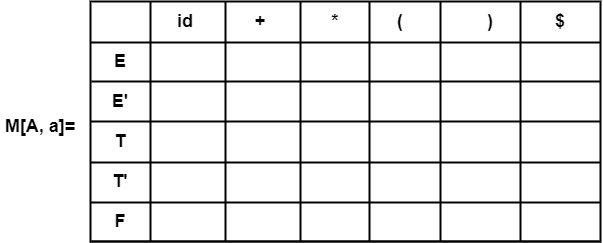# Construct a Predictive Parsing table for the following grammar & also check whether string id + id * id is accepted or not.

Problem − Consider the following grammar −

E → TE′

E′ → +TE′|ε

T′ → FT′

T′ → FT′|ε

F → (E)|id

Solution −

Step1− Elimination of Left Recursion & perform Left Factoring

As there is no left recursion in Grammar so, we will proceed as it is. Also, there is no need for Left Factoring.

Step2− Computation of FIRST

FIRST(E) = FIRST(T) = FIRST(F) = {(, id}

FIRST (E′) = {+, ε}

FIRST (T′) = {*, ε}

Step3− Computation of FOLLOW

FOLLOW (E) = FOLLOW(E′) = {), $} FOLLOW (T) = FOLLOW(T′) = {+, ),$}

FOLLOW (F) = {+,*,),$} Step4− Construction of Predictive Parsing Table Create the table, i.e., write all non-terminals row-wise & all terminal column-wise.Now, fill the table by applying rules from the construction of the Predictive Parsing Table. • E → TE′ Comparing E → TE′with A → α  E → TE′ A → Α ∴ A = E, α = TE′ ∴ FIRST(α) = FIRST(TE′) = FIRST(T) = {(, id} Applying Rule (1) of Predictive Parsing Table ∴ ADD E → TE′ to M[E, ( ] and M[E, id] ∴ write E → TE′in front of Row (E)and Columns {(, id} (1) • E′ → +TE′|𝛆 Comparing it with A → α  E → +TE′ A → α ∴ A = E′ α = +TE′ ∴ FIRST(α) = FIRST(+TE′) = {+} ∴ ADD E → +TE′ to M[E′, +] ∴ write production E′ → +TE′ in front of Row (E′)and Column (+) (2) • E′ → ε Comparing it with A → α  E → ε A → α ∴ α = ε ∴ FIRST(α) = {ε} ∴ Applying Rule (2)of the Predictive Parsing Table. Find FOLLOW (E′) = { ),$}

∴ ADD Production E′ → ε to M[E′, )] and M[E′, $] ∴ write E′ → ε in front of Row (E′)and Column {$, )}                                                         (3)

• T → FT′

Comparing it with A → α

 T → FT′ A → α

∴ A = T, α = FT′

∴ FIRST(α) = FIRST (FT′) = FIRST (F) = {(, id}

∴ ADD Production T → FT′ to M[T, (] and M[T, id]

∴ write T → FT′ in front of Row (T)and Column {(, id}                                                       (4)

• T′ →*FT′

Comparing it with A → α

 T → *FT′ A → α

∴ FIRST(α) = FIRST (* FT′) = {*}

∴ ADD Production T → +FT′ to M[T′,*]

∴ write T′ →∗ FT′ in front of Row (T′)and Column {*} (5)

• T′ → ε

Comparing it with A → α

 T′ → ε A → α

∴ A = T′

α = ε

∴ FIRST(α) = FIRST {𝜀} = {ε}

∴ Applying Rule (2)of the Predictive Parsing Table.

Find FOLLOW (A) = FOLLOW (T′) = {+, ), $} ∴ ADD T′ → ε to M[T′, +], M[T′, )] and M[T′,$]

∴ write T′ → ε in front of Row (T′)and Column {+, ), $} (6) • F →(E) Comparing it with A → α  F → (E) A → A ∴ A = F, α = E ∴ FIRST(α) = FIRST ((E)) = {(} ∴ ADD F → (E) to M[F, (] ∴ write F → (E) in front of Row (F)and Column (( ) (7) • F → id Comparing it with A → α  F → Id A → A ∴ FIRST(α) = FIRST (id) = {id} ∴ ADD F → id to M[F, id] ∴ write F → id in front of Row (F)and Column (id) (8) Combining all statements (1) to (8) will generate the following Predictive or LL (1) Parsing Table −  Id + * ( )$ E E → TE′ E → TE′ E′ E′ → +TE′ E′ → ε T′ → ε T T → FT′ T → FT′ T′ T′ → ε T′ →* FT′ T′ → ε T′ → ε F F → id F → (E)

Step5− Checking Acceptance of String id + id * id using Predictive Parsing Program

Initially, the stack will contain the starting symbol E and $at the bottom of the stack. Input Buffer will contain a string attached with$ at the right end.

If the top of stack = Current Input Symbol, then symbol from the top of the stack will be popped, and also input pointer advances or reads next symbol.

The sequence of Moves by Predictive Parser

Stack Input Action
$Eid + id * id$E → TE′
$E′Tid + id * id$T → FT′
$E′T′Fid + id * id$F → id
$E′T′idid + id * id$Remove id
$E′T′+id * id$T′ → ε
$E′+id * id$E′ → +TE′
$E′T ++id * id$Remove +
$E′Tid * id$T → FT′
$E′T′Fid * id$F → id
$E′T′idid * id$Remove id
$E′T′* id$T′ →* FT′
$E′T′F ** id$Remove *
$E′T′Fid$F → id
$E′T′idid$Remove id
$E′T′$T′ → ε
$E′$E′ → ε
$E$Accept